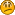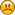## Weapon Employment Zone

Started by 375CT, June 06, 2014, 12:17:55 AM

#### 375CT

#15
Quote from: mman on June 27, 2014, 06:20:11 AM
Quote from: 375CT on June 26, 2014, 11:44:10 PM
Well, so far the original questions remains, is Litz's WEZ (a term taken from Air Combat for budget error analysis) flat or not?That's easy one. It must be normal distribution. No sense to use flat distribution, that would lead to strange results.

Makes sense, but how to be sure? I get and agree to the point you made, but making no sense, well, that's the hard part out of this, because we are not dealing with any way to test the actual implementation.

http://ballistipedia.com/index.php?title=Main_Page

I understand you are recomending going CEP for a more realistic simulation, am I right on my assumption?

#### mman

#16
Quote from: 375CT on June 27, 2014, 08:36:48 AM
Makes sense, but how to be sure? I get and agree to the point you made, but making no sense, well, that's the hard part out of this, because we are not dealing with any way to test the actual implementation.

Litz speaks about standard deviations when he mentions distribution factors. This alone tells us that probability distribution can't be flat. Another proof is that litz's tool gives approx. same results as mine. And mine is based on normal distributions.

Quote from: 375CT on June 27, 2014, 08:36:48 AM
I understand you are recomending going CEP for a more realistic simulation, am I right on my assumption?
No, I'm only recommending CEP for group size measuring. For wez tool you can use monte carlo or CEP depending which properties you value. I decided to go with CEP because for me it was more important to compare different calibers, bullets and conditions than get absolutely accurate results in hitting probability. Problem with monte carlo is that you get slightly different results for every calculation. That's not desirable if you try to compare something.

In practise you can never accurately calculate hitting probability anyway. That's simply because you don't know exact standard daviations for distribution factors. WEZ tool is always at it's best for comparing not for absolute probabilities.

#### 375CT

#17
Quote from: mman on June 27, 2014, 09:20:58 AM
Quote from: 375CT on June 27, 2014, 08:36:48 AM
Makes sense, but how to be sure? I get and agree to the point you made, but making no sense, well, that's the hard part out of this, because we are not dealing with any way to test the actual implementation.

Litz speaks about standard deviations when he mentions distribution factors. This alone tells us that probability distribution can't be flat. Another proof is that litz's tool gives approx. same results as mine. And mine is based on normal distributions.

Quote from: 375CT on June 27, 2014, 08:36:48 AM
I understand you are recomending going CEP for a more realistic simulation, am I right on my assumption?
No, I'm only recommending CEP for group size measuring. For wez tool you can use monte carlo or CEP depending which properties you value. I decided to go with CEP because for me it was more important to compare different calibers, bullets and conditions than get absolutely accurate results in hitting probability. Problem with monte carlo is that you get slightly different results for every calculation. That's not desirable if you try to compare something.

In practise you can never accurately calculate hitting probability anyway. That's simply because you don't know exact standard daviations for distribution factors. WEZ tool is always at it's best for comparing not for absolute probabilities.

Mman, thanks for the tips, they are much appreciated for sure.

#18
1) My random number generator generates the same output sequence for the same seed.
2) My random number generator is not recalculated if somewhere else on the spreadsheet an (unlinked) cell is recalculated.

#### mman

#19
Robert can you explain input values of your random number generators?

#20
From Bfx_help(...)
90   ==========================Non volatile random numbers===========================
91   i is a positive integer number, b is the bottom value, t (>b) is the top value
92   <r> is an optional range
93   random_number = BfX_Ran(i; <r>) 0 <= random_number <= 1]
94   b <= random_number <= t = BfX_Ranb(i; b; t; <r>)
95   c and s are center and width of normal distribution
96   normal distribution b <= random_number <= t= BfX_Rang(i; c; s; b; t; <r>)
97   use <r> to link cells to guide Excels calculation order

i is the seed.

<r> is a cell or a range

B   C   D
2   seed   1234567
3
4   0,051118901   =BfX_Ran(seed)
5   0,207298019   =BfX_Ran(seed;C4)
6   0,529684412   =BfX_Ran(seed;C5)
7   0,164931189   =BfX_Ran(seed;C6)
8   0,760708209   =BfX_Ran(seed;C7)
9   0,711878956   =BfX_Ran(seed;C8)
10   0,434484066   =BfX_Ran(seed;C9)
11   0,032066679   =BfX_Ran(seed;C10)
12   0,018770791   =BfX_Ran(seed;C11)
13   0,197369616   =BfX_Ran(seed;C12)
14
15   bfx_ran    refers to the previous cell in order to force
16      a specific order of random number (cell) processing

My random number is non volatile.
Volatile means according to MS:
Excel supports the concept of a volatile function, that is, one whose value cannot be assumed to be the same from one moment to the next even if none of its arguments (if it takes any) has changed. Excel reevaluates cells that contain volatile functions, together with all dependents, every time that it recalculates. For this reason, too much reliance on volatile functions can make recalculation times slow. Use them sparingly.

The following Excel functions are volatile:

NOW

TODAY

RAND

OFFSET

INDIRECT

INFO (depending on its arguments)

CELL (depending on its arguments)

#### mman

#21
Thanks Robert! I made another WEZ tool based on monte carlo using your bfx_Rang random number.

Input values:CEP based WEZ versus monte carlo based WEZ. Results for 4 different bullets/conditions.+/- values for monte carlo are to show 95 % confidence interval which means that 95 % of the time error is less than that. To get that narrow confidence interval I had to calculate 100 000 simulations per bullet per range. It took 92 minutes while CEP tool did the same (and other calculations as well) in 7 sec. However CEP tool seems to give errors of 0 - 7 percentage points. As expected CEP tool is still most of the time good enough for comparison.

#22
Cool.

would it be possible to include the effects of a  hold over/under (and the horizontal equivalent)? (in combination with cant)

#### 375CT

#23
Mman,

Is this WEZ tool inlcuded in your Hit Prob spreadsheet? Asking because I'd like to ry it if you don't mind.

LIked the idea of going Monte Carlo for the random sequence then using CEP for hit prob. Nice touch.

I know, you guys are Excel experts, now I'm going to have to do some VB.net and see what I can come up with.

Not quite clear the difference in the MV fields errors, any more detail will be kindly grateful.

#### mman

#24
Quote from: admin on June 30, 2014, 08:08:28 PM
would it be possible to include the effects of a hold over/under (and the horizontal equivalent)? (in combination with cant)

This input field allows you to move group center in relation to target, if that is what you meant?Quote from: 375CT on June 30, 2014, 08:55:22 PM
Is this WEZ tool inlcuded in your Hit Prob spreadsheet? Asking because I'd like to ry it if you don't mind.
Yes, it is. I'll attach it later today.

Quote from: 375CT on June 30, 2014, 08:55:22 PM
LIked the idea of going Monte Carlo for the random sequence then using CEP for hit prob. Nice touch.
That's not the case. I calculated hit probability with two different methods:
1. CEP which is nothing to do with random sequence. Basicly it is just analytical way to express hit probability on round target.
2. Monte carlo which is based on Robert's rang.

Quote from: 375CT on June 30, 2014, 08:55:22 PM
Not quite clear the difference in the MV fields errors, any more detail will be kindly grateful.
On dispersion factors field you define SD of group (all values are expressed in standard deviations). On group center bias field you define how far away in average group center is from target center.
e.g. "Muzzle velocity variation" of 3 m/s means that your MV SD is 3 m/s. "Wrong muzzle velocity" of 3 m/s means that you have assumed muzzle velocity to be 3 m/s higher than it actually is. --> Your ballistic calculation gives you wrong holdover/scope correction clicks which leads to group center shift downwards.

#25
Quote from: mman on July 01, 2014, 09:12:33 AM

This input field allows you to move group center in relation to target, if that is what you meant?

Yes. I our case, at 300m, people aim with their telecopes at a white sticker 20cm below the electronic target center.

#### mman

#26
Quote from: admin on July 01, 2014, 10:44:34 AM
Quote from: mman on July 01, 2014, 09:12:33 AM

This input field allows you to move group center in relation to target, if that is what you meant?

Yes. I our case, at 300m, people aim with their telecopes at a white sticker 20cm below the electronic target center.
But I guess aiming error is compensated with equivalent scope correction so that group center is on correct place?

Most of it is still in finnish, sorry.

#### 375CT

#27
Mman,

Great work, really liked the new sheet. Sorry if I miswrote my question, but I meant that MC was just for creating the random sampling, then applying CEP to yield the HP in one of the calcs you did. Please correct me if I'm wrong.

Got it regarding the variations and SD inputs.

Any time later, english comments would be nice, my finnish is awful#### 375CT

#28
In case you guys need to go VB.net some day here is a useful RNG (Gaussian) snippet I tested and works really well. The original code was written in C# which I later converted.

Public Class RandomGenerator

'indicates that an extra deviates was already calculated
Private _hasAnotherDeviate As Boolean
'The other deviate calculated using the Box-Muller transformation
Private _otherGaussianDeviate As Double
Public Sub New()
Me.New(New Random())
End Sub

Public Sub New(ByVal seed As Integer)
Me.New(New Random(seed))
End Sub

Public Sub New(ByVal random As Random)
_random = random
End Sub

' returns a normally distributed deviate with zero mean and unit variance.
' Adapted from Numerical Recipe page 289: Normal (Gaussian) Deviates

Public Function NormalDeviate() As Double
Dim rsq, v1, v2 As Double
If _hasAnotherDeviate Then
'we have an extra deviate handy. Reset the flag and return it
_hasAnotherDeviate = False
Return _otherGaussianDeviate
End If
Do
v1 = UniformDeviate(-1, 1) 'pick two uniform number
v2 = UniformDeviate(-1, 1) 'in the square extending from -1 to +1
rsq = v1 * v1 + v2 * v2 'see if they are in the unit circle
Loop While rsq >= 1.0 OrElse rsq = 0.0
'now make the box-muller transformation to get two normal deviates.
Dim fac As Double = Math.Sqrt(-2.0 * Math.Log(rsq) / rsq)
'Return one and save one for next time
_otherGaussianDeviate = v1 * fac
_hasAnotherDeviate = True
Return v2 * fac
End Function

' Returns a uniformly distributed random number between min and max.
Public Function UniformDeviate(ByVal min As Double, ByVal max As Double) As Double
Return (max - min) * _random.NextDouble() + min
End Function

' Returns a random number between 0 and 1
Public Function NextDouble() As Double
Return _random.NextDouble()
End Function

End Class